# Algebra DefinitionsPage 1

#### WATCH ALL SLIDES

Slide 1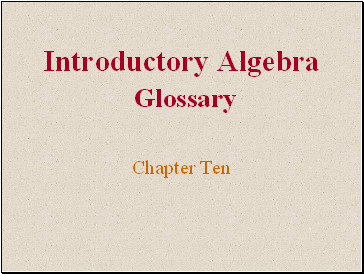Introductory Algebra Glossary

Chapter Ten

Slide 2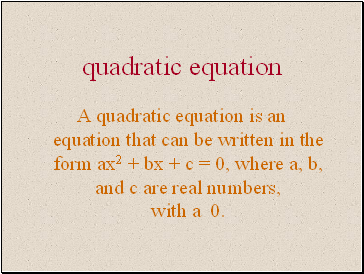A quadratic equation is an equation that can be written in the form ax2 + bx + c = 0, where a, b, and c are real numbers, with a 0.

Slide 3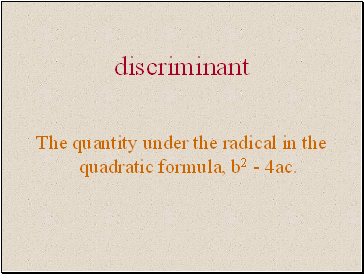## Discriminant

The quantity under the radical in the quadratic formula, b2 - 4ac.

Slide 4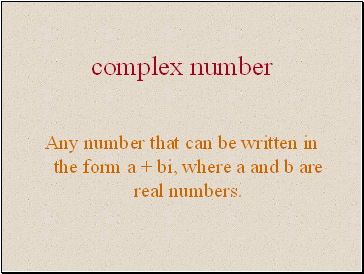## Complex number

Any number that can be written in the form a + bi, where a and b are real numbers.

Slide 5## Imaginary number

A complex number a + bi

with b  0.

Slide 6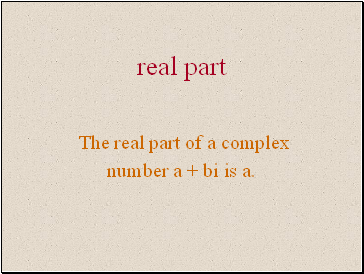## Real part

The real part of a complex

number a + bi is a.

Slide 7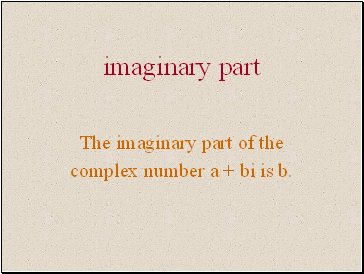## Imaginary part

The imaginary part of the

complex number a + bi is b.

Slide 8## Standard form of a complex number

The standard form of a

complex number is a + bi.

Slide 9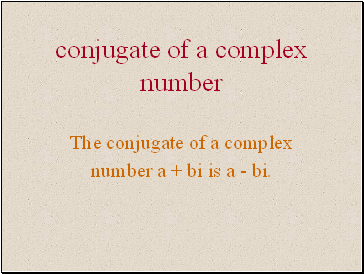## Conjugate of a complex number

The conjugate of a complex

number a + bi is a - bi.

Slide 10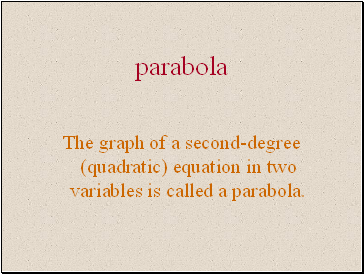## Parabola

The graph of a second-degree (quadratic) equation in two variables is called a parabola.

Slide 11parabola

The graph of a second-degree (quadratic) equation in two variables is called a parabola.

Slide 12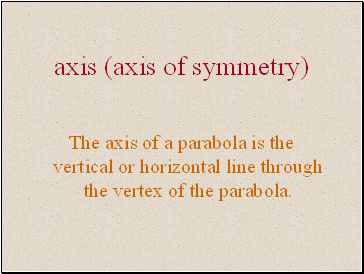## Axis (axis of symmetry)

The axis of a parabola is the vertical or horizontal line through the vertex of the parabola.

Slide 13A function defined by an equation of the form f(x) = ax2 + bx + c, for real numbers a, b, and c, with a 0.

Slide 14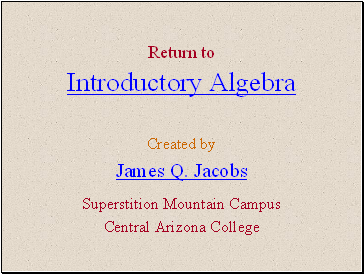Created by

James Q. Jacobs

Superstition Mountain Campus

Central Arizona College